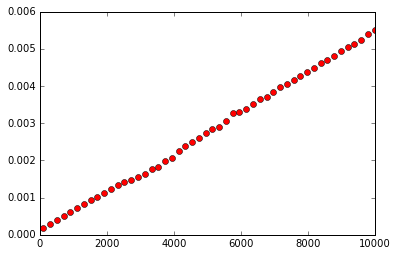1. Motives
2. Objectives
3. Mechanisms

## 1. Motives¶

In :
%matplotlib inline
import matplotlib.pyplot as plt
import numpy as np
from timeit import timeit

def time_array_front_insert_delete(n):
return timeit('lst.insert(0, None) ; del lst',
'lst = list(range({}))'.format(n),
number=1000)

ns = np.linspace(100, 10000, 50)
plt.plot(ns, [time_array_front_insert_delete(int(n)) for n in ns], 'ro')
plt.show()Problem: arrays are not efficient for insertions & deletions (except for at the end).

... and these types of operations can be critical for structures where, say, some internal ordering must be preserved while adding elements (e.g., a database whose records are sorted/searchable by key)

In :
# consider:

def concatenate(arr1, arr2):
"""Concatenates the contents of arr1 and arr2 as efficiently (time-wise)
as possible, so that the resulting structure can be used to index all
combined elements (arr1's followed by arr2's)."""

# option 1:
for x in arr2:
arr1.append(x)
return arr1

# option 2:
arr1.extend(arr2)
return arr1

# option 3:
return arr1 + arr2


All options for concatenate are = $O(n)$, where $n$ is the number of elements involved.

Problem: all elements must be contiguous (in memory) in an array, as they are indexed from a common base address. No simple way to "bridge" the contents of two disjoint arrays --- i.e., no way to make arr1[len(arr1)] somehow "jump" to arr2.

... this makes it so separately allocated, large structures cannot be merged or uniformly indexed efficiently (without first performing an expensive "defragmentation" operation to physically move the structures next to each other).

## 2. Objectives¶

We need a new data storage mechanism for constructing data structures that:

• does not require monolithic, contiguous memory allocation,
• allows individual elements to be flexibly and efficiently reorganized,
• and preserves the ability to locate (e.g., via position) and iterate over elements

## 3. Mechanisms¶

### 3.1. Two-Element Lists¶

In :
# data items
i1 = 'lions'
i2 = 'tigers'
i3 = 'bears'
i4 = 'oh, my'

In :
link1 = [i1, None]

In :
link1 = link2

In :
def link_iter(head):

In :
for i in link_iter(link1):
print(i)

lions
tigers
bears
oh, my

In :
link0 = ['walruses', link1]

In :
for i in link_iter(link0):
print(i)

walruses
lions
tigers
bears
oh, my

In :
link2 = ['goats', link2]

In :
for i in link_iter(link0):
print(i)

walruses
lions
tigers
goats
bears
oh, my


In :
class Link:
def __init__(self, val, next=None):
self.val = val
self.next = next

In :
head = Link(i4)

In :
def linked_list_iter(lst):
while lst:
yield lst.val
lst = lst.next

In :
for i in linked_list_iter(linked_lst):
print(i)

lions
tigers
bears
oh, my

In :
# recursive implementation (note: recursive call obviates need for the loop)
if lst:
yield lst.val

In :
for i in linked_list_iter(linked_lst):
print(i)

lions
tigers
bears
oh, my

In :
class LinkedList:
def __init__(self):

def prepend(self, val):

def __iter__(self):
while cursor:
yield cursor.val
cursor = cursor.next

def __repr__(self):
return '[' + ', '.join(str(x) for x in self) + ']'

In :
lst = LinkedList()
for i in range(10):
lst.prepend(i)

In :
lst

Out:
[9, 8, 7, 6, 5, 4, 3, 2, 1, 0]
In :
lst2 = LinkedList()
for i in range(10, 20):
lst2.prepend(i)

while p.next: # advance p to last node in lst2
p = p.next


In :
lst2

Out:
[19, 18, 17, 16, 15, 14, 13, 12, 11, 10, 9, 8, 7, 6, 5, 4, 3, 2, 1, 0]
In :
class BinaryLink:
def __init__(self, val, left=None, right=None):
self.val = val
self.left = left
self.right = right

In :
root = BinaryLink(10)

In :
def tree_iter(root):
if root:
yield root.val
yield from tree_iter(root.left)
yield from tree_iter(root.right)

In :
for i in tree_iter(root):
print(i)

10
20
15
30
45

In :
class NaryLink:
def __init__(self, val, n=2):
self.val = val
self.children = [None] * n

def __getitem__(self, idx):
return self.children[idx]

def __setitem__(self, idx, val):
self.children[idx] = val

def __iter__(self):
for c in self.children:
yield c

In :
root = NaryLink('Kingdoms', n=5)

def tree_iter(root):
if root:
yield root.val
for c in root:
yield from tree_iter(c)

In :
for x in tree_iter(root):
print(x)

Kingdoms
Animalia
Plantae
Fungi
Chytridiomycota